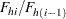### Sequential Random Sampling

If you specify the option METHOD=SEQ and do not include a SIZE statement, PROC SURVEYSELECT uses the equal probability version of Chromy’s method for sequential random sampling. This method selects units sequentially with equal probability and without replacement. See Chromy (1979) and Williams and Chromy (1980) for details. See the section PPS Sequential Sampling for a description of Chromy’s PPS selection method.

Sequential random sampling controls the distribution of the sample by spreading it throughout the sampling frame or stratum, thus providing implicit stratification according to the order of units in the frame or stratum. You can use the CONTROL statement to sort the input data set by the CONTROL variables before sample selection. If you also use a STRATA statement, PROC SURVEYSELECT sorts by the CONTROL variables within strata. By default (or if you specify the SORT=SERP option), the procedure uses hierarchic serpentine ordering for sorting. If you specify the SORT=NEST option, the procedure uses nested sorting. See the section Sorting by CONTROL Variables for descriptions of serpentine and nested sorting. If you do not specify a CONTROL statement, PROC SURVEYSELECT applies sequential selection to the observations in the order in which they appear in the input data set.

Following Chromy’s method of sequential selection, PROC SURVEYSELECT randomly chooses a starting unit from the entire stratum (or frame, if the design is not stratified). With this unit as the first one, the procedure treats the stratum units as a closed loop. This is done so that all pairwise (joint) selection probabilities are positive and an unbiased variance estimator can be obtained. The procedure numbers units sequentially from the random start to the end of the stratum and then continues from the beginning of the stratum until all units are numbered.

Beginning with the randomly chosen starting unit, PROC SURVEYSELECT accumulates the expected number of selections (hits), where the expected number of selections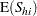equals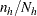for all unitsin stratum. The procedure computes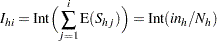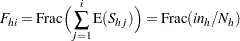where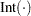denotes the integer part of the number, and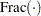denotes the fractional part.

Considering each unit sequentially, Chromy’s method determines whether unitis selected by comparing the total number of selections for the first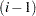units,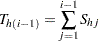with the value of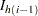.

If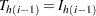, Chromy’s method determines whether or not unitis selected as follows. If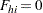or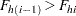, then unitis selected with certainty. Otherwise, unitis selected with probability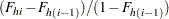If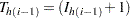, Chromy’s method determines whether or not unitis selected as follows. Ifor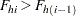, then the unit is not selected. Otherwise, unitis selected with probability